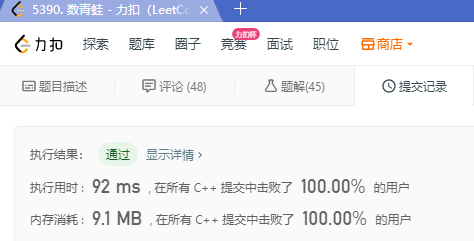# LeetCode 1419. 数青蛙（脑洞题）

1576 篇文章 40 订阅

## 1. 题目

示例 1：

1 <= croakOfFrogs.length <= 10^5



## 2. 解题

• c, r, o, a, k任意时刻字符数量，按这个次序非升
• 最后时候数量必须相等
• 5个字符在任意时刻的最大值为需要的青蛙数量
• 5个字符满了一只青蛙，则减去它，后面可以重复使用这只青蛙。
class Solution {
public:
int minNumberOfFrogs(string croakOfFrogs) {
int i, frog=0, c, r, o, a, k;
c=r=o=a=k=i=0;
for(i = 0; i < croakOfFrogs.size(); ++i)
{
if(croakOfFrogs[i]=='c') c++;
else if(croakOfFrogs[i]=='r') r++;
else if(croakOfFrogs[i]=='o') o++;
else if(croakOfFrogs[i]=='a') a++;
else k++;
if(!(c>=r&&r>=o&&o>=a&&a>=k)) return -1;//次序不对
if(i == croakOfFrogs.size()-1 && !(a==r&&r==o&&o==a&&a==k))
return -1;//最后不相等
frog = max(frog,max(c,max(r,max(o,max(a,k)))));
if(c&&r&&o&&a&&k)//满了一只
c--,r--,o--,a--,k--;//减去它
}
return frog;
}
};©️2020 CSDN 皮肤主题: Age of Ai 设计师:meimeiellie点击重新获取扫码支付1.余额是钱包充值的虚拟货币，按照1:1的比例进行支付金额的抵扣。
2.余额无法直接购买下载，可以购买VIP、C币套餐、付费专栏及课程。余额充值